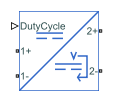# Average-Value DC-DC Converter

Average-value DC-DC converter

• Library:
• Simscape / Electrical / Semiconductors & Converters / Converters

•## Description

The Average-Value DC-DC Converter block represents a controlled average-value DC-DC converter. You can program the block as a buck converter, boost converter, or buck-boost converter by providing the duty cycle. The diagram shows the equivalent circuit for the block with duty cycle as input. The converter contains a controlled current source and a controlled voltage source. Use the duty cycle, the current reference, or the voltage reference ports as control input to convert the electrical energy between the connected components on either side of the converter.### Equations

If you set the Control input parameter to ```Duty cycle```, the input current and output voltage are a function of the duty cycle and depend on the converter type.

Voltage and Current Equations

Converter TypeOutput Voltage, ${v}_{2}$Input Current, ${i}_{1}$
Buck${v}_{2}=DutyCycle\cdot {v}_{1}$${i}_{1}=DutyCycle\cdot {i}_{2}$
Boost${v}_{2}=\frac{{v}_{1}}{1-DutyCycle}$${i}_{1}=\frac{{i}_{2}}{1-DutyCycle}$
Buck-Boost${v}_{2}=\frac{DutyCycle\cdot {v}_{1}}{1-DutyCycle}$${i}_{1}=\frac{DutyCycle\cdot {i}_{2}}{1-DutyCycle}$

If you set the Control input parameter to ```Current reference```, the converter sets the output current and it computes the voltage.

Similarly, if you set the Control input parameter to `Voltage reference`, the converter sets the output voltage and it computes the current.

### Limitations and Assumptions

• The input voltage is positive.

• All converter types use the same polarity for input and output.

## Ports

### Conserving

expand all

Electrical conserving port associated with the duty cycle.

#### Dependencies

To enable this port, set Control Input to ```Duty Cycle```.

Data Types: `double`

Electrical conserving port associated with the current reference.

#### Dependencies

To enable this port, set Control Input to ```Current reference```.

Data Types: `double`

Electrical conserving port associated with the voltage reference.

#### Dependencies

To enable this port, set Control Input to ```Voltage reference```.

Data Types: `double`

Electrical conserving port associated with the positive terminal of the first DC voltage.

Data Types: `double`

Electrical conserving port associated with the negative terminal of the first DC voltage.

Data Types: `double`

Electrical conserving port associated with the positive terminal of the second DC voltage.

Data Types: `double`

Electrical conserving port associated with the negative terminal of the second DC voltage.

Data Types: `double`

## Parameters

expand all

Specify the control input to convert the electrical energy between the two sides.

Type of converter.

#### Dependencies

To enable this parameter, set Control Input to ```Duty Cycle```.

Specify the parameterization of the converter efficiency. If you select `Tabulated`, conduction losses will depend on the provided output current.

Note

For tabulated efficiency, the extrapolation method is nearest. Therefore, the efficiency does not go above the highest value or below the lowest value in the Efficiency vector (%) parameter.

Efficiency of the converter, in percentage.

#### Dependencies

To enable this parameter, set Converter efficiency to `Constant`.

Vector of output currents.

#### Dependencies

To enable this parameter, set Converter efficiency to `Tabulated`.

Vector of efficiencies for each output current specified in Output current vector, in percentage. This parameter must have the same size of Output current vector.

#### Dependencies

To enable this parameter, set Converter efficiency to `Tabulated`.

## Version History

Introduced in R2018b